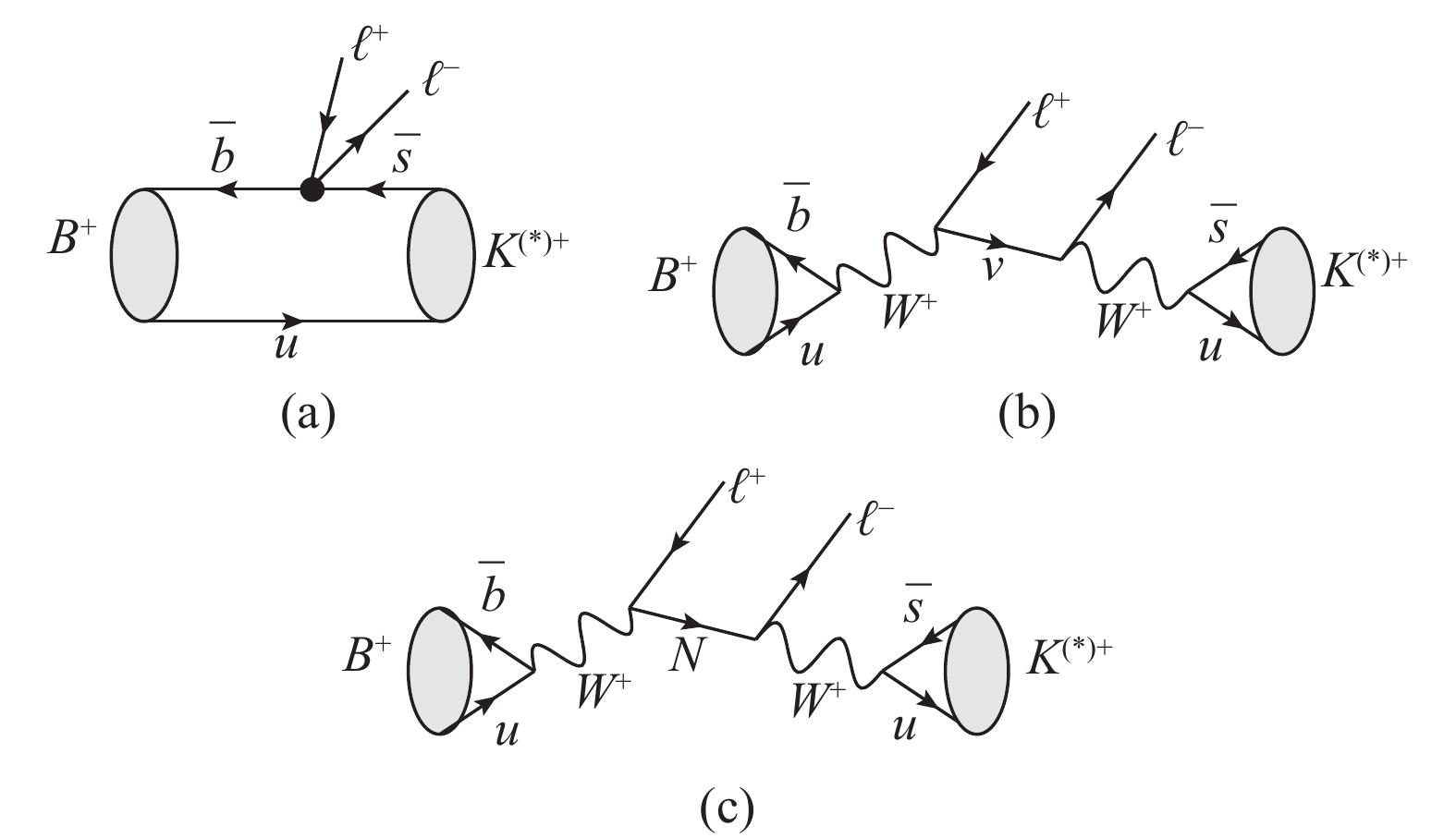# Study of the Standard Model and Majorana neutrino contributions to ${ B}^{{ +}} { \to} { K}^{{ (*){ \pm}}}{ \mu}^{ +}{ \mu}^{{ \mp}}$• Lepton number violation processes can be induced by the Majorana neutrino exchange, which provide evidence for the Majorana nature of neutrinos. In addition to the natural explanation of the small neutrino masses, Type-I seesaw mechanism predicts the existence of Majorana neutrinos. The aim of this work is to study the B meson rare decays $B^{+} \to K^{(*)+}\mu^+\mu^-$in the Standard Model and its extensions, and then to investigate the same-sign decay process $B^{+}\to K^{(*)-}\mu^{+}\mu^+$. The corresponding dilepton invariant mass distributions are calculated. It is found that the dilepton angular distributions could shed light on the properties of new interactions induced by Majorana neutrinos.
••Get Citation
Hong-Lei Li, Peng-Cheng Lu, Cong-Feng Qiao, Zong-Guo Si and Ying Wang. Study of the Standard Model and Majorana neutrino contributions to ${ B}^{{ +}} { \to} { K}^{{ (*){ \pm}}}{ \mu}^{ +}{ \mu}^{{ \mp}}$[J]. Chinese Physics C, 2019, 43(2): 1-1. doi: 10.1088/1674-1137/43/2/023101
Hong-Lei Li, Peng-Cheng Lu, Cong-Feng Qiao, Zong-Guo Si and Ying Wang. Study of the Standard Model and Majorana neutrino contributions to ${ B}^{{ +}} { \to} { K}^{{ (*){ \pm}}}{ \mu}^{ +}{ \mu}^{{ \mp}}$[J]. Chinese Physics C, 2019, 43(2): 1-1.Milestone
Received: 2018-09-30
Article Metric

Article Views(557)
PDF Downloads(38)
Cited by(0)
Policy on re-use
To reuse of Open Access content published by CPC, for content published under the terms of the Creative Commons Attribution 3.0 license (“CC CY”), the users don’t need to request permission to copy, distribute and display the final published version of the article and to create derivative works, subject to appropriate attribution.
###### 通讯作者: 陈斌, bchen63@163.com
• 1.

沈阳化工大学材料科学与工程学院 沈阳 110142

Title:
Email:

## Study of the Standard Model and Majorana neutrino contributions to ${ B}^{{ +}} { \to} { K}^{{ (*){ \pm}}}{ \mu}^{ +}{ \mu}^{{ \mp}}$• 1. School of Physics and Technology, University of Jinan, Jinan Shandong 250022, China
• 2. School of Physics, Shandong University, Jinan, Shandong 250100, China
• 3. School of Physical Sciences, University of Chinese Academy of Sciences, YuQuan Road 19A, Beijing 100049, China
• 4. CAS Center for Excellence in Particle Physics, Beijing 100049, China
• 5. School of Science, Beijing University of Civil Engineering and Architecture, Beijing 100044, China

Abstract: Lepton number violation processes can be induced by the Majorana neutrino exchange, which provide evidence for the Majorana nature of neutrinos. In addition to the natural explanation of the small neutrino masses, Type-I seesaw mechanism predicts the existence of Majorana neutrinos. The aim of this work is to study the B meson rare decays $B^{+} \to K^{(*)+}\mu^+\mu^-$in the Standard Model and its extensions, and then to investigate the same-sign decay process $B^{+}\to K^{(*)-}\mu^{+}\mu^+$. The corresponding dilepton invariant mass distributions are calculated. It is found that the dilepton angular distributions could shed light on the properties of new interactions induced by Majorana neutrinos.

### HTML1.   Introduction2.   Theoretical framework3.   Numerical analysis
Reference (62)
PDF查看关注分享

Top

### 目录/DownLoad:  Full-Size Img  PowerPoint